Name:    Chapter 5 Test

Multiple Choice
Identify the choice that best completes the statement or answers the question.

Write the ratio in simplest form.

1.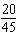a.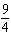c.b.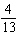d.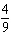2.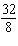a.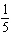c.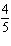b.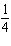d.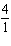3.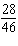a.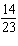c.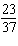b.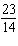d.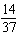4.

You use the punch recipe to create a larger batch that uses 6 liters of ginger ale. How many cans of pineapple juice should you use?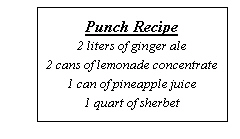a. 3 c. 12 b. 1 d. 6

Write a unit rate for the situation.

5.

\$60 spent in 3 trips
 a.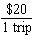c.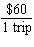b.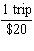d.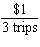Find the distance.

6.

d  ?  , r = 41 m/sec, t = 5 sec
 a. 204 m/sec c. 205 m/sec b. 205 m d. 204 m

Find how far the object travels in the given amount of time.

7.

18 hours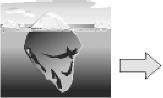Moves 2 meters every 3 hours.
 a. 36 m c. 12 m b. 27 m d. 8 m

8.

45 seconds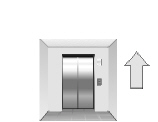Rises 5 stories every 9 seconds.
 a. 81 stories c. 24 stories b. 25 stories d. 225 stories

9.

42 seconds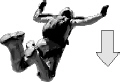Falls 2280 feet every 12 seconds.
 a. 8,000 ft c. 27,360 ft b. 7,955 ft d. 7,980 ft

Find the mean of the data.

10.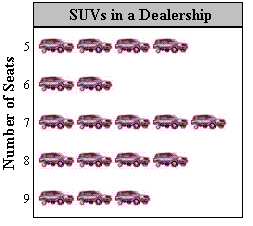a. 5.8 c. 3.6 b. 8.2 d. 7

Numeric Response

1.

A space shuttle travels 280 miles per minute. What is the rate, in miles per hour?

2.

You read 7 pages in 3 minutes. How many pages can you read in 6 hours?

Describe the “average” of the data.

1.

Players on a team: 12, 13, 13, 13, 13, 13, 14

2.

A data set has a mean of 9, a median of 15, and a mode of 8. Which of the numbers 9, 15, and 8 must be in the data set? Explain.